# 【AI实战】训练第一个AI模型：MNIST手写数字识别模型

2018/08/11 00:41MNIST是一个经典的手写数字数据集，来自美国国家标准与技术研究所，由不同人手写的0至9的数字构成，由60000个训练样本集和10000个测试样本集构成，每个样本的尺寸为28x28，以二进制格式存储，如下图所示：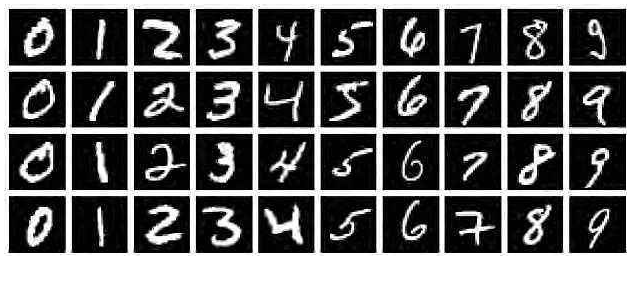MNIST手写数字识别模型的主要任务是：输入一张手写数字的图像，然后识别图像中手写的是哪个数字。

0、AI建模主要步骤【注意】由于MNIST数据集太经典了，很多深度学习书籍在介绍该入门模型案例时，基本上就是直接下载获取数据，然后就进行模型训练，最后得出一个准确率出来。但这样的入门案例学习后，当要拿自己的数据来训练模型，却往往不知该如何处理数据、如何训练、如何应用。在本文，将分两种情况进行介绍：（1）使用MNIST数据（本案例），（2）使用自己的数据。

1、准确数据

（1）使用MNIST数据（本案例）
MNIST数据集由于非常经典，已集成在tensorflow里面，可以直接加载使用，也可以从MNIST的官网上（http://yann.lecun.com/exdb/mnist/） 直接下载数据集，代码如下：

from tensorflow.examples.tutorials.mnist import input_data

# 数据集路径
data_dir='/home/roger/data/work/tensorflow/data/mnist'

# 自动下载 MNIST 数据集

# 如果自动下载失败，则手工从官网上下载 MNIST 数据集，然后进行加载
# 下载地址  http://yann.lecun.com/exdb/mnist/
#mnist=input_data.read_data_sets(data_dir,one_hot=True)

（2）使用自己的数据

① 整个文件打标签。例如MNIST数据集，每个图像只有1个数字，可以从0至9建10个文件夹，里面放相应数字的图像；也可以定义一个规则对图像进行命名，如按标签+序号命名；还可以在数据库里面创建一张对应表，存储文件名与标签之间的关联关系。如下图：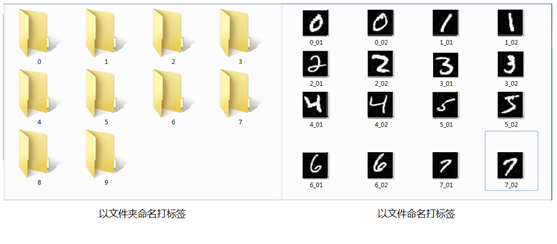② 圈定区域打标签。例如ImageNet的物体识别数据集，由于每张图片上有各种物体，这些物体位于不同位置，因此需要圈定某个区域进行标注，目前比较流行的是VOC2007、VOC2012数据格式，这是使用xml文件保存图片中某个物体的名称（name）和位置信息（xmin,ymin,xmax,ymax）。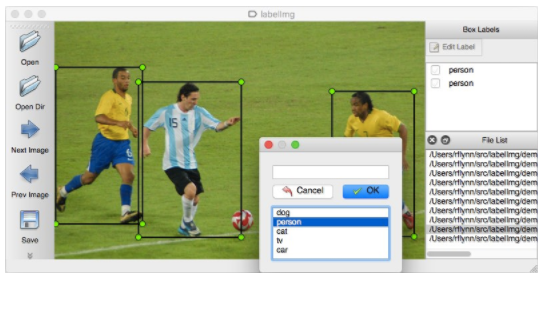③ 数据截段打标签。针对语音识别、文字识别等，有些是将数据截成一段一段的语音或句子，然后在另外的文件中记录对应的标签信息。

2、数据预处理

（1）使用MNIST数据（本案例）

（2）使用自己的数据a. 统一格式：即统一基础数据的格式，例如图像数据集，则全部统一为jpg格式；语音数据集，则全部统一为wav格式；文字数据集，则全部统一为UTF-8的纯文本格式等，方便模型的处理；
b. 调整尺寸：根据模型的输入要求，将样本数据全部调整为统一尺寸。例如LeNet模型是32x32，AlexNet是224x224，VGG是224x224等；
c. 灰度化：根据模型需要，有些要求输入灰度图像，有些要求输入RGB彩色图像；
d. 去噪平滑：为提升输入图像的质量，对图像进行去噪平滑处理，可使用中值滤波器、高斯滤波器等进行图像的去噪处理。如果训练数据集的图像质量很好了，则无须作去噪处理；
e. 其它处理：根据模型需要进行直方图均衡化、二值化、腐蚀、膨胀等相关的处理；
f. 样本增强：有一种观点认为神经网络是靠数据喂出来的，如果能够增加训练数据的样本量，提供海量数据进行训练，则能够有效提升算法的质量。常见的样本增强方式有：水平翻转图像、随机裁剪、平移变换，颜色、光照变换等，如下图所示：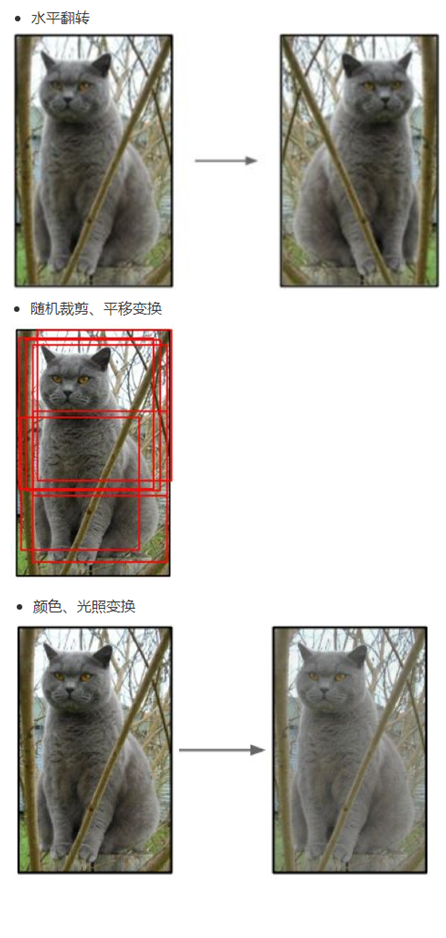3、划分数据集

（1）使用MNIST数据（本案例）

# 提取训练集、测试集
train_xdata = mnist.train.images
test_xdata = mnist.test.images

# 提取标签数据
train_labels = mnist.train.labels
test_labels = mnist.test.labels

（2）使用自己的数据

from sklearn.cross_validation import train_test_split

# 随机选取75%的数据作为训练样本，其余25%的数据作为测试样本
# X_data：数据集
# y_labels：数据集对应的标签
X_train,X_test,y_train,y_test=train_test_split(X_data,y_labels,test_size=0.25,random_state=33)

4、配置模型

（1）选择模型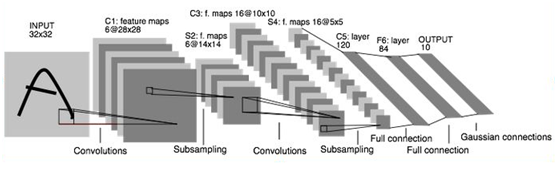（2）设置参数

step_cnt=10000    # 训练模型的迭代步数
batch_size = 100    # 每次迭代批量取样本数据的量
learning_rate = 0.001    # 学习率

5、训练模型

（1）构建模型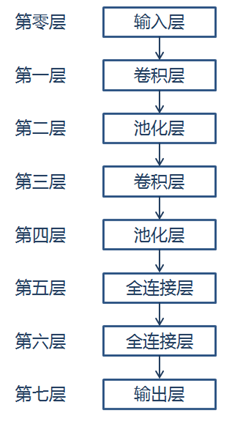# 训练数据，占位符
x = tf.placeholder("float", shape=[None, 784])
# 训练的标签数据，占位符
y_ = tf.placeholder("float", shape=[None, 10])
# 将样本数据转为28x28
x_image = tf.reshape(x, [-1, 28, 28, 1])

# 保留概率，用于 dropout 层
keep_prob = tf.placeholder(tf.float32)

# 第一层：卷积层
# 卷积核尺寸为5x5，通道数为1，深度为32，移动步长为1，采用ReLU激励函数
conv1_weights = tf.get_variable("conv1_weights", [5, 5, 1, 32], initializer=tf.truncated_normal_initializer(stddev=0.1))
conv1_biases = tf.get_variable("conv1_biases", , initializer=tf.constant_initializer(0.0))
conv1 = tf.nn.conv2d(x_image, conv1_weights, strides=[1, 1, 1, 1], padding='SAME')

# 第二层：最大池化层
# 池化核的尺寸为2x2，移动步长为2，使用全0填充
pool1 = tf.nn.max_pool(relu1, ksize=[1, 2, 2, 1], strides=[1, 2, 2, 1], padding='SAME')

# 第三层：卷积层
# 卷积核尺寸为5x5，通道数为32，深度为64，移动步长为1，采用ReLU激励函数
conv2_weights = tf.get_variable("conv2_weights", [5, 5, 32, 64], initializer=tf.truncated_normal_initializer(stddev=0.1))
conv2_biases = tf.get_variable("conv2_biases", , initializer=tf.constant_initializer(0.0))
conv2 = tf.nn.conv2d(pool1, conv2_weights, strides=[1, 1, 1, 1], padding='SAME')

# 第四层：最大池化层
# 池化核尺寸为2x2, 移动步长为2，使用全0填充
pool2 = tf.nn.max_pool(relu2, ksize=[1, 2, 2, 1], strides=[1, 2, 2, 1], padding='SAME')

# 第五层：全连接层
fc1_weights = tf.get_variable("fc1_weights", [7 * 7 * 64, 1024],
initializer=tf.truncated_normal_initializer(stddev=0.1))
fc1_baises = tf.get_variable("fc1_baises", , initializer=tf.constant_initializer(0.1))
pool2_vector = tf.reshape(pool2, [-1, 7 * 7 * 64])
fc1 = tf.nn.relu(tf.matmul(pool2_vector, fc1_weights) + fc1_baises)

# Dropout层（即按keep_prob的概率保留数据，其它丢弃），以防止过拟合
fc1_dropout = tf.nn.dropout(fc1, keep_prob)

# 第六层：全连接层
fc2_weights = tf.get_variable("fc2_weights", [1024, 10],
initializer=tf.truncated_normal_initializer(stddev=0.1))  # 神经元节点数1024, 分类节点10
fc2_biases = tf.get_variable("fc2_biases", , initializer=tf.constant_initializer(0.1))
fc2 = tf.matmul(fc1_dropout, fc2_weights) + fc2_biases

# 第七层：输出层
y_conv = tf.nn.softmax(fc2)

（2）训练模型

# 定义交叉熵损失函数
# y_ 为真实标签
cross_entropy = tf.reduce_mean(-tf.reduce_sum(y_ * tf.log(y_conv), reduction_indices=))

# 选择优化器，使优化器最小化损失函数

# 返回模型预测的最大概率的结果，并与真实值作比较
correct_prediction = tf.equal(tf.argmax(y_conv, 1), tf.argmax(y_, 1))

# 用平均值来统计测试准确率
accuracy = tf.reduce_mean(tf.cast(correct_prediction, tf.float32))

# 训练模型
saver=tf.train.Saver()
with tf.Session() as sess:
tf.global_variables_initializer().run()

for step in range(step_cnt):
batch = mnist.train.next_batch(batch_size)
if step % 100 == 0:
# 每迭代100步进行一次评估，输出结果，保存模型，便于及时了解模型训练进展
train_accuracy = accuracy.eval(feed_dict={x: batch, y_: batch, keep_prob: 1.0})
print("step %d, training accuracy %g" % (step, train_accuracy))
saver.save(sess,model_dir+'/my_mnist_model.ctpk',global_step=step)
train_step.run(feed_dict={x: batch, y_: batch, keep_prob: 0.8})

# 使用测试数据测试准确率
print("test accuracy %g" % accuracy.eval(feed_dict={x: mnist.test.images, y_: mnist.test.labels, keep_prob: 1.0}))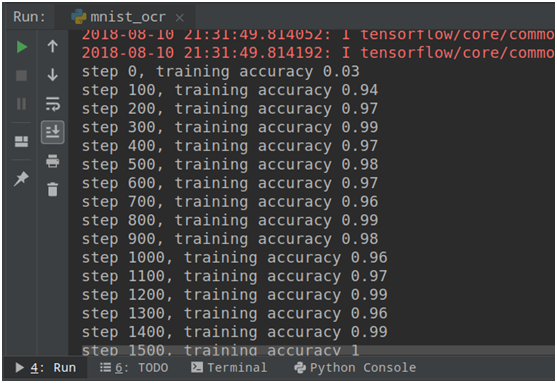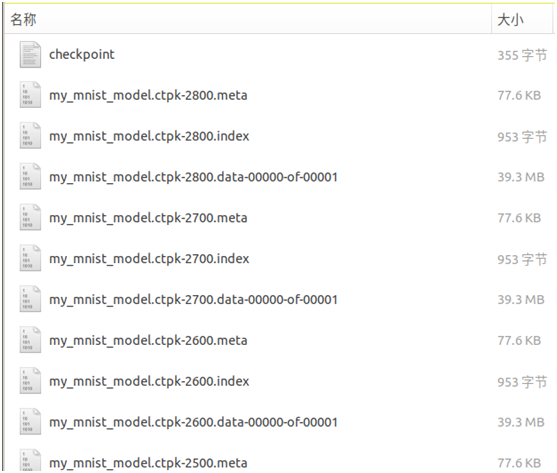6、评估优化

# 使用测试数据测试准确率
test_acc=accuracy.eval(feed_dict={x: test_xdata, y_: test_labels, keep_prob: 1.0})
print("test accuracy %g" %test_acc)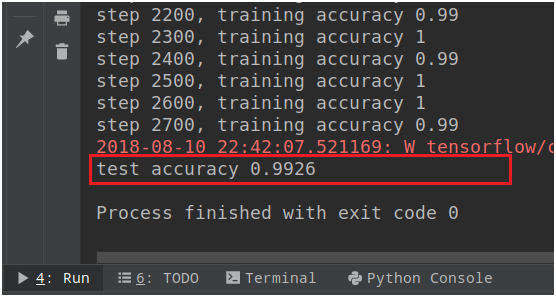7、模型应用

# 加载 MNIST 模型
saver = tf.train.Saver()
with tf.Session() as sess:
saver.restore(sess, tf.train.latest_checkpoint(model_dir))

# 随机提取 MNIST 测试集的一个样本数据和标签
test_len=len(mnist.test.images)
test_idx=random.randint(0,test_len-1)
x_image=mnist.test.images[test_idx]
y=np.argmax(mnist.test.labels[test_idx])

# 跑模型进行识别
y_conv = tf.argmax(y_conv,1)
pred=sess.run(y_conv,feed_dict={x:[x_image], keep_prob: 1.0})

print('正确：',y,'，预测：',pred)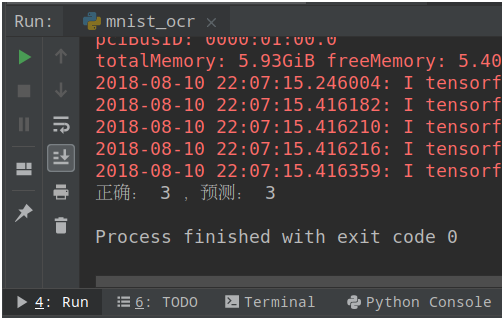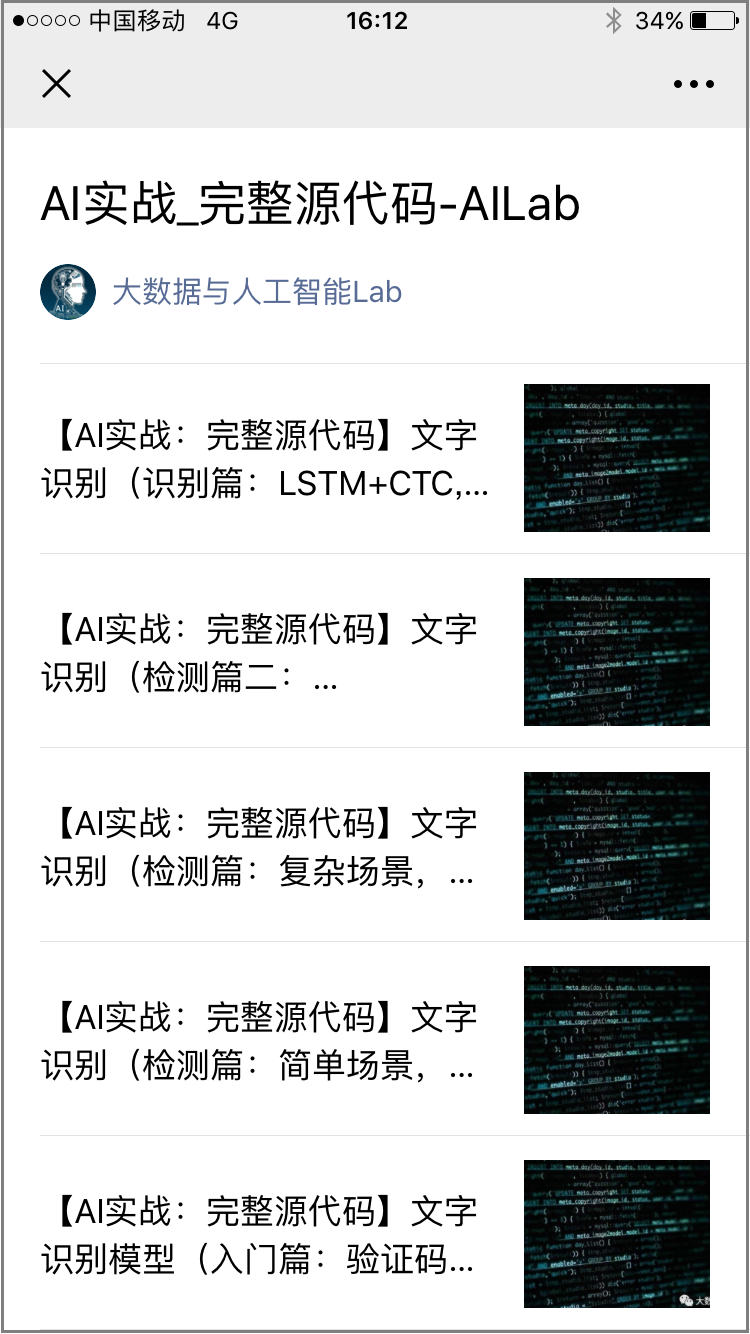•### 作者的其它热门文章

1
16 收藏

1 评论
16 收藏
1